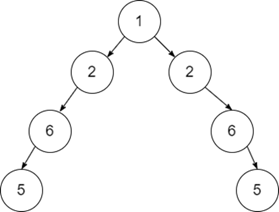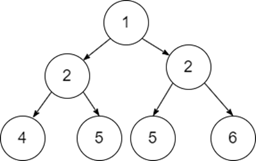# Symmetric Tree in C++

C++Server Side ProgrammingProgramming

Let us suppose we have a binary tree and the task is to check whether it constructs a symmetry of itself or not. A Symmetric Binary tree constructs the mirror image of itself.

For Example

Input-1:Output:

True

Explanation:

Since the given binary tree constructs the mirror image of itself, the output is True.

Input-2:Output:

False

Explanation:

Since the given binary tree doesn't make a mirror image of itself, it is not symmetric.

## Approach to Solve this Problem

A symmetric binary tree is a tree which is the mirror image of itself, which means we have to check whether the left and right nodes of the tree are the same or not.

A Boolean function will initially check for the left node and the right node. If the nodes are empty or NULL, then it will return True. For other cases, we will check if it has left or right children, then it must be the same so that it will be a Symmetric Tree.

• Take a Binary Tree which contains the root and its children.
• A Boolean helper function helper(node*root1, node*root2) takes two roots of the same tree which helps to check whether the left child and the right child are the same or not.
• If the tree is empty or NULL, then we will return True.
• Check recursively whether the left node and the right node of the tree is equal or not.
• Return False if all the above conditions are not satisfying.

## Example

Live Demo

#include<bits/stdc++.h>
using namespace std;
struct treenode {
int data;
treenode * left;
treenode * right;
};
struct treenode * createNode(int d) {
struct treenode * root = new treenode;
root -> data = d;
root -> left = NULL;
root -> right = NULL;
return root;
}
bool helper(struct treenode * root1, struct treenode * root2) {
if (root1 == NULL and root2 == NULL)
return true;
if (root1 and root2 and root1 -> data == root2 -> data)
return (helper(root1 -> left, root2 -> right) and helper(root1 -> right, root2 -> left));
return false;
}
bool isSymmetry(struct treenode * root) {
return helper(root, root);
}
int main() {
struct treenode * root = NULL;
root = createNode(4);
root -> left = createNode(2);
root -> right = createNode(2);
root -> left -> right = createNode(7);
root -> left -> left = createNode(5);
root -> right -> left = createNode(5);
root -> right -> right = createNode(7);
if (isSymmetry(root)) {
cout << "True" << endl;
} else {
cout << "False" << endl;
}
return 0;
}

Running the above code will generate the output as,

## Output

False

Explanation:

Since the given tree is not symmetric, we get the output as False.